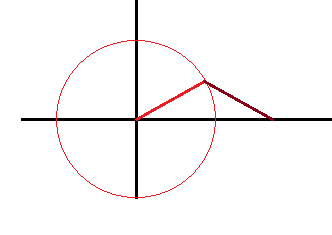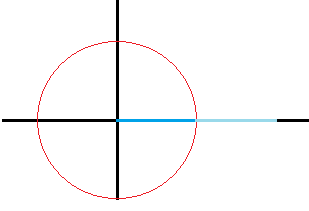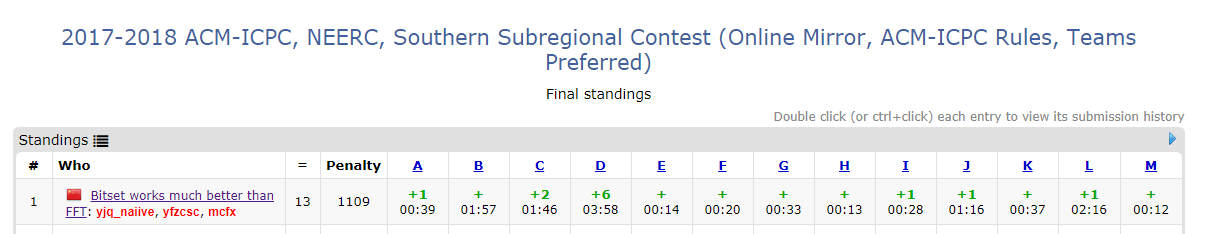2018/12/14 17:34

# 信号, 集合, 多项式, 以及卷积性变换

[TOC]

## 卷积

• 多项式乘法?
• 狄利克雷卷积?

2\left( \sum_{i=0}^{\text{len}(P)-1}S^2_{x+i}P^2_i \right) + \left( \sum_{i=0}^{\text{len}(P)-1}S_{x+i}P_i^3 \right) \end{align} $$现在它变成了三个看上去像卷积的东西... 然而我们非常滑稽地发现: 这个卷积中下标运算不是加法而是减法! 咋办? 我们只需要用下面这个小学生都知道的式子:$$ a-b=a+(-b) $$我们想办法把其中一个串的下标变成负的! 然而直接变成负的的话好像非常不清真. 我们再加个 \text{len} 把它补成正的吧! 于是我们就要搞一个这样的变换来预处理:$$ P'i=P{\text{len}(P)-i} $$emmmmm... 这不就是™ std::reverse 了一下么 (也就是说当你发现减法卷积的时候翻转其中一个就可以了) 然后就可以搞事情了! 我们预处理出 S_i^2,S_i^3,P_i^2,P_i^3 然后再 std::reverse 一下 P, 卷起来看哪位是 0 就可以了! 因为都是整数, NTT/FFT均可. ### 一点优化? 刚刚我们的做法要算三个卷积做⑨遍FFT/NTT, 常数直接起飞. 如果原串和模板串都带通配符的话并没有别的办法, 只能像个⑨一样做⑨遍FFT. (讲道理⑨怎么会FFT呢) (实际上我们本质上是多项式乘法再加法的形式, 所以按照点值表示的运算法则算就可以了, 总计 7 遍 FFT) 但是如果只有模板串或只有原串带通配符呢? 我们可以考虑复数! 刚刚我们为啥不能直接做差呢? 因为正负相消, 两个不匹配位置可能一个 +k 一个 -k 造成假匹配. 如果是复数呢? 我们按照惯例先说个结论: k 个模长为 1 的复数相加, 它们的和为 k 当且仅当这些复数都是 1. 于是我们把这个差搬到 e^i 的指数上就好辣!$$ \begin{align} M(x)&=\sum_{k=0}^{\text{len}(P)-1}e^{\frac{i\pi(S_{x+k}-P_k)}\Sigma} \ &=\sum_{k=0}^{\text{len}(P)-1}e^{\frac{i\pi S_{x+k}}\Sigma}e^{\frac{-i\pi P_k}\Sigma} \end{align}  为啥?(大雾

## 写在最后

0
0 收藏

### 作者的其它热门文章0 评论
0 收藏
0